# Math Worksheets Land

Math Worksheets For All Ages

# Math Worksheets Land

Math Worksheets For All Ages

# Long Division Worksheets

When we are trying to determine the quotient of larger numbers, we use a technique called long division. This follow the same method of solving division-based operations it is just setup differently to accommodate all the extra integers and keep you well organized throughout the process of solving the operation. It begins by placing the dividend in a division box and placing the divisor on the outside of it. A full walk through of the long division process can be found below in both the lesson and the complete tutorial found at the bottom of this page. The worksheets will give students plenty of practice to make sure they get the hang of this new technique.

### Aligned Standard: Grade 5 Base Ten - 5.NBT.6

• Answer Keys - These are for all the unlocked materials above.

### Homework Sheets

No remainders are left behind in these bad boys.

• Homework 1 - Lots of expanded division problems on this sheet.
• Homework 2 - Count how many times you subtracted 8. You subtracted 8 seven times.
• Homework 3 - A well leveled problem for you.

### Practice Worksheets

I used several different mixed formats with each sheet to make sure that students understand that a quotient is a quotient regardless of how it is set up.

• Practice 1 - It can be very helpful to remember to place a comma to denote place value in very large numbers.
• Practice 2 - Think of the divisor as a block. How many blocks will it take you to build the number that is being divided?
• Practice 3 - Division can be seen as a form of extended subtraction. Each time you can subtract a divisor from a dividend, it's worth one.

### Math Skill Quizzes

Just setting up these problems properly for calculation might be a task for some students.

• Quiz 1 - If the dividend looks a bit overwhelming, subtract the divisor a few times to get the number that you would like to worth with. Remember each time you subtract equal 1 to the value of the quotient.
• Quiz 2 - Knowing mental math can be a lifesaver for long division. Make sure that you know facts to 12 like the back of your hand.
• Quiz 3 - Using regular lined paper can be very helpful when setting up these problems. The lines will help you make sense of the setting of each problem.

### Long Division Tips

The concept of this well used operation is somehow related to getting a good grip on algebra and performing high level of mathematical problems. Long division is a more advanced segment in the use of and understanding of this operation. It is a key method for solving or finding the answer or remainders of complex or large numbers. Understanding how this thing works will help you to solve complex division problems within minutes. If you want to learn the tricks, follow these basic steps.

1. Identify the Dividend and Divisor - First, identify the dividend and divisor on a piece of paper. Place the dividend inside the division box and divisor outside the box. The top part of the division is for the quotient or your answer.

2. Fit the Divisor into The Dividend - The division will take place from left to right. Start with the first digit. If the first is less than the divisor add the second digit or third digit to make it greater than the divisor. Once you find a value the divisor fits into, drop the remainder and drop subsequent values to the end of the remainder.

3. Multiplying Divisor - Start multiplying the divisor with the dividend's digit or digits and write the nearest answer under the digit. Write the quotient on top.

4. Subtract - Find the difference of the two numbers and place the next number down with the answer. Divide further following the same method. Mention all the quotients on the top of box. They are your final answer.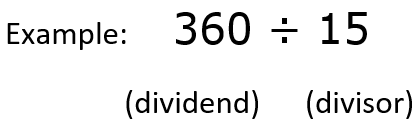Step 1) Set it Up - Place the dividend insider the division box and the divisor outside of it.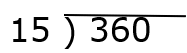Step 2) Work the Divisor Through the Dividend - a) The first digit 3 is divided by the divisor (15) to reveal 0. So we move to the tens place (36).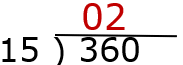b) 15 goes into 36, 2 times and leaves a remainder of 6. We drop that remainder down below and subtract out the value, as displayed below.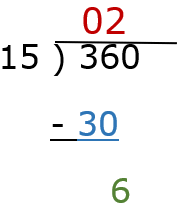c) We drop the zero from the ones place of the dividend to that remainder and see how many times the divisor goes into that.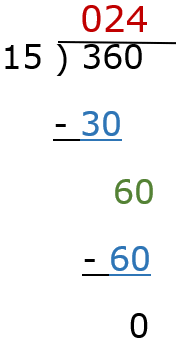## The Grid Method

This one of those topics that teachers do not look forward to tackling with their students.It is mostly due to the fact that it requires a large helping of skills to do well.The majority of those skills were learned in previous grade years.One technique that I find works well with teaching students this skill is the grid method. It is a good way to stay organized throughout the course of processing these operations. This is not a mental approach to the problem, but as the name indicates, it encourages the use of a grid. The grid encases and separates each number of the dividend.The divisor is placed just outside and to the left of the grid that is formed around each number of the dividend.From there proceed with working the divisor into each single integer of the dividend from left to right. When we are working the operations on a particular integer in the dividend, we keep those actions confined to that one box.We place the values we have pulled out at the top of the boxes. We carry down any remainder values and process this all the way through to completion.Using this method will help you stay much more organized than the traditional approach to these problems. Here is an example of how you set a long division problem into a division box with the grid method setup around it. This will help you stay organized and can pay off.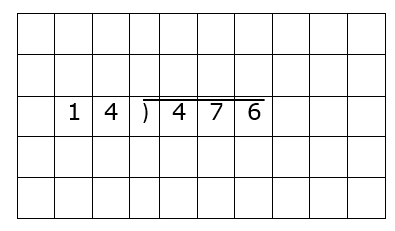Unlock all the answers, worksheets, homework, tests and more!
Save Tons of Time! Make My Life Easier Now

## Thanks and Don't Forget To Tell Your Friends!

I would appreciate everyone letting me know if you find any errors. I'm getting a little older these days and my eyes are going. Please contact me, to let me know. I'll fix it ASAP.